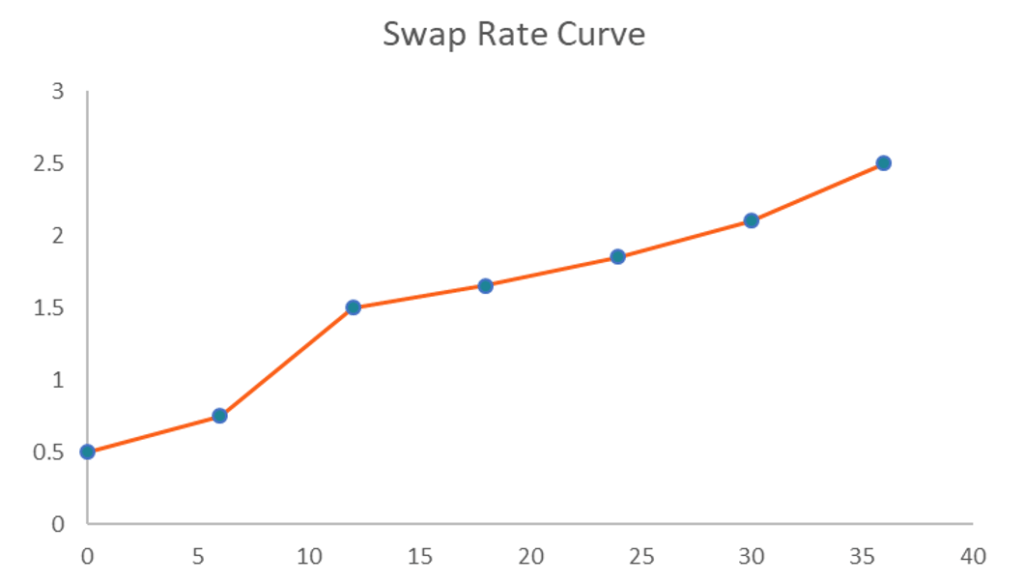# Swap Rate Curve

A chart that depicts the relationship between swap rates and available maturities

## What is the Swap Rate Curve?

The swap rate curve is a chart that depicts the relationship between swap rates and all available corresponding maturities. Essentially, it indicates the expected returns of the swap on different maturity dates. The swap rate curve can be considered as a swap’s equivalent of a bond’s yield curve, and is a par curve as it is based on par swaps.The swap rate curve is a two-dimensional plot drawn against x-axis and y-axis. The swap rates are plotted against the y-axis while the times to maturity are drawn on the x-axis. Generally, the curve is upward sloping since long-term swap rates tend to be higher than short-term rates.

### Understanding the Swap Rate Curve

The swap rate curve is frequently drawn together with the yield curve of a government bond (e.g., US Treasury securities). In some cases, the two curves may coincide. However, the swap rate curve and the bond’s yield curve often diverge from each other. If the two curves diverge from each other, the swap spread is presented. Historical data show that the swap rate curve tended to be above the yield curve, thus indicating positive swap spreads.

The swap rate curve is extensively used in financial markets. For example, it is frequently used as a benchmark for interest rates. In addition, the curve can be utilized to assess the market participants’ sentiment regarding the prevailing market conditions (particularly risk).

### How to Create the Swap Rate Curve in Excel?

The swap rate curve can be easily created using Microsoft Excel. It can be done by following the steps below:

1. Create a table that will contain the necessary information, including the swap rates and corresponding maturity dates.
2. In the first column, list the swap rates.
3. List the corresponding maturities in the second column.
4. Using the mouse or keyboard, highlight the created table. Then, press Insert->Recommended Charts->All Charts->Line Chart and choose the chart with the single line. Alternatively, you can do it in the following way by pressing Insert->Scatter->Scatter with Straight Lines and Markers. Your swap rate curve must look like the chart on the image above.

### Swap Curve and Valuation

Swap contracts are traded in Over-The-Counter Markets (OTC). As a swap contract has two legs to it, we must must determine the present value of both legs to determine the value of the swap contract. An interest rate swap contract for example, the fixed leg for example is fairly simple, as future cash flows are set at inception. The floating leg requires a more complex calculation as it is subject to change with the fluctuation of interest rates. The forward rate curve must be used to determine the forward rate of each future floating payment.

More Resources

CFI offers the Financial Modeling & Valuation Analyst (FMVA)™ certification program for those looking to take their careers to the next level. To keep learning and advancing your career, the following resources will be helpful:

• Excel for Finance
• Swap